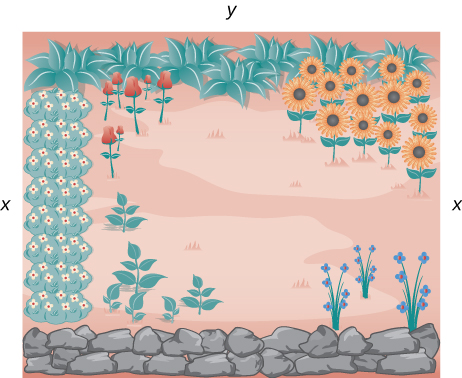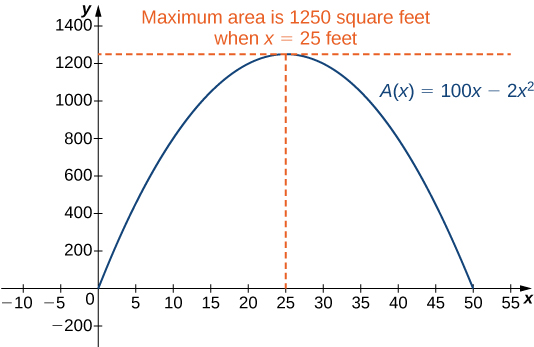# 4.7 Applied optimization problems

 Page 1 / 8
• Set up and solve optimization problems in several applied fields.

One common application of calculus is calculating the minimum or maximum value of a function. For example, companies often want to minimize production costs or maximize revenue. In manufacturing, it is often desirable to minimize the amount of material used to package a product with a certain volume. In this section, we show how to set up these types of minimization and maximization problems and solve them by using the tools developed in this chapter.

## Solving optimization problems over a closed, bounded interval

The basic idea of the optimization problems    that follow is the same. We have a particular quantity that we are interested in maximizing or minimizing. However, we also have some auxiliary condition that needs to be satisfied. For example, in [link] , we are interested in maximizing the area of a rectangular garden. Certainly, if we keep making the side lengths of the garden larger, the area will continue to become larger. However, what if we have some restriction on how much fencing we can use for the perimeter? In this case, we cannot make the garden as large as we like. Let’s look at how we can maximize the area of a rectangle subject to some constraint on the perimeter.

## Maximizing the area of a garden

A rectangular garden is to be constructed using a rock wall as one side of the garden and wire fencing for the other three sides ( [link] ). Given $100$ ft of wire fencing, determine the dimensions that would create a garden of maximum area. What is the maximum area?We want to determine the measurements x and y that will create a garden with a maximum area using 100 ft of fencing.

Let $x$ denote the length of the side of the garden perpendicular to the rock wall and $y$ denote the length of the side parallel to the rock wall. Then the area of the garden is

$A=x·y.$

We want to find the maximum possible area subject to the constraint that the total fencing is $100\phantom{\rule{0.2em}{0ex}}\text{ft}.$ From [link] , the total amount of fencing used will be $2x+y.$ Therefore, the constraint equation is

$2x+y=100.$

Solving this equation for $y,$ we have $y=100-2x.$ Thus, we can write the area as

$A\left(x\right)=x·\left(100-2x\right)=100x-2{x}^{2}.$

Before trying to maximize the area function $A\left(x\right)=100x-2{x}^{2},$ we need to determine the domain under consideration. To construct a rectangular garden, we certainly need the lengths of both sides to be positive. Therefore, we need $x>0$ and $y>0.$ Since $y=100-2x,$ if $y>0,$ then $x<50.$ Therefore, we are trying to determine the maximum value of $A\left(x\right)$ for $x$ over the open interval $\left(0,50\right).$ We do not know that a function necessarily has a maximum value over an open interval. However, we do know that a continuous function has an absolute maximum (and absolute minimum) over a closed interval. Therefore, let’s consider the function $A\left(x\right)=100x-2{x}^{2}$ over the closed interval $\left[0,50\right].$ If the maximum value occurs at an interior point, then we have found the value $x$ in the open interval $\left(0,50\right)$ that maximizes the area of the garden. Therefore, we consider the following problem:

Maximize $A\left(x\right)=100x-2{x}^{2}$ over the interval $\left[0,50\right].$

As mentioned earlier, since $A$ is a continuous function on a closed, bounded interval, by the extreme value theorem, it has a maximum and a minimum. These extreme values occur either at endpoints or critical points. At the endpoints, $A\left(x\right)=0.$ Since the area is positive for all $x$ in the open interval $\left(0,50\right),$ the maximum must occur at a critical point. Differentiating the function $A\left(x\right),$ we obtain

${A}^{\prime }\left(x\right)=100-4x.$

Therefore, the only critical point is $x=25$ ( [link] ). We conclude that the maximum area must occur when $x=25.$ Then we have $y=100-2x=100-2\left(25\right)=50.$ To maximize the area of the garden, let $x=25$ ft and $y=50\phantom{\rule{0.2em}{0ex}}\text{ft}.$ The area of this garden is $1250{\phantom{\rule{0.2em}{0ex}}\text{ft}}^{2}.$To maximize the area of the garden, we need to find the maximum value of the function A ( x ) = 100 x − 2 x 2 .

any genius online ? I need help!!
Pina
need to learn polynomial
Zakariya
i will teach...
nandu
I'm waiting
Zakariya
evaluate the following computation (x³-8/x-2)
teach me how to solve the first law of calculus.
what is differentiation
f(x) = x-2 g(x) = 3x + 5 fog(x)? f(x)/g(x)
fog(x)= f(g(x)) = x-2 = 3x+5-2 = 3x+3 f(x)/g(x)= x-2/3x+5
diron
pweding paturo nsa calculus?
jimmy
how to use fundamental theorem to solve exponential
find the bounded area of the parabola y^2=4x and y=16x
what is absolute value means?
Chicken nuggets
Hugh
🐔
MM
🐔🦃 nuggets
MM
(mathematics) For a complex number a+bi, the principal square root of the sum of the squares of its real and imaginary parts, √a2+b2 . Denoted by | |. The absolute value |x| of a real number x is √x2 , which is equal to x if x is non-negative, and −x if x is negative.
Ismael
find integration of loge x
find the volume of a solid about the y-axis, x=0, x=1, y=0, y=7+x^3
how does this work
Can calculus give the answers as same as other methods give in basic classes while solving the numericals?
log tan (x/4+x/2)
Rohan
Rohan
y=(x^2 + 3x).(eipix)
Claudia
Ismael
A Function F(X)=Sinx+cosx is odd or even?
neither
David
Neither
Lovuyiso
f(x)=1/1+x^2 |=[-3,1]
apa itu?
fauzi
determine the area of the region enclosed by x²+y=1,2x-y+4=0
Hi
MP
Hi too
Vic
hello please anyone with calculus PDF should share
Which kind of pdf do you want bro?
Aftab
hi
Abdul
can I get calculus in pdf
Abdul
explain for me
Usman
okay I have such documents
Fitzgerald
Hamza

#### Get Jobilize Job Search Mobile App in your pocket Now!By Richley CrapoBy OpenStaxBy Brooke DelaneyBy Nick SwainBy John GabrieliBy Janet ForresterBy Christine ZeelieBy Brooke DelaneyBy Edgar DelgadoBy OpenStax